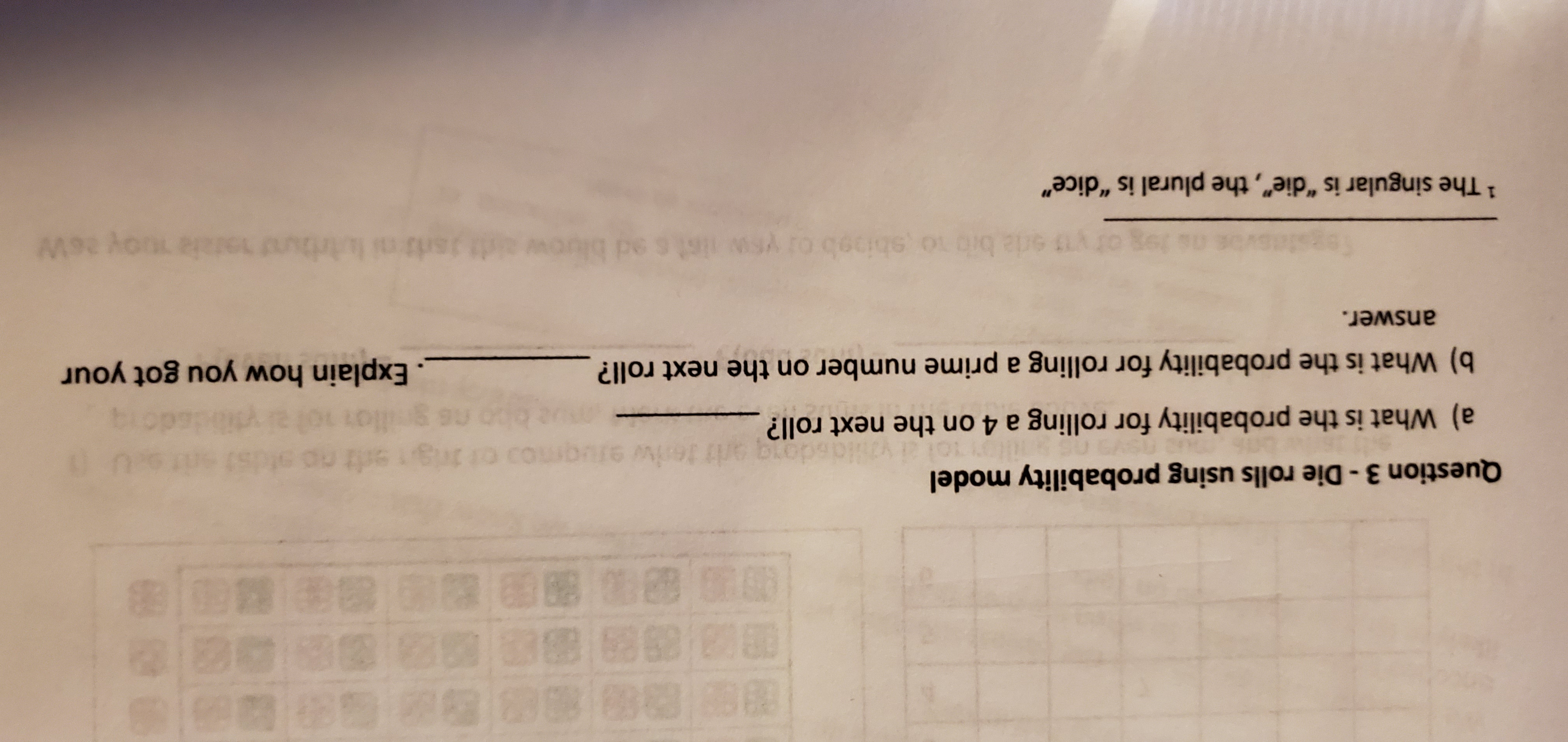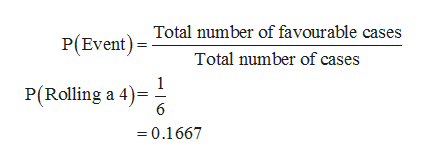# B63/89 89 88 882 00 88 9 Bae aBB asQuestion 3-Die rolls using probability modeloigedt no0 gsdongiwvsa) What is the probability for rolling a 4 on the next roll?Explain how you got yourb) What is the probability for rolling a prime number on the next roll?answer.s2utlaysd2 bib o sbioob or ysw ilst s ed bluow it26W19 ot.YEW1 The singular is "die", the plural is "dice"

Question
3 viewshelp_outlineImage TranscriptioncloseB 63/89 89 88 8 82 00 88 9 Bae aB B as Question 3-Die rolls using probability model oigedt no 0 g sdong iw vs a) What is the probability for rolling a 4 on the next roll? Explain how you got your b) What is the probability for rolling a prime number on the next roll? answer. s2 utlay sd2 bib o sbioob or ysw ilst s ed bluow it 26W 19 ot. YEW 1 The singular is "die", the plural is "dice" fullscreen
check_circle

Step 1

a)

The probability for rolling a 4 on the next roll:

It is given that die is rolled one time.

When a six sided die is rolled one time, the probability of each outcome is 1/6.

The sample space when a die is rolled is {1, 2, 3, 4, 5, and 6} and all outcomes are equally likely.

The sample space contains the value 4. Thus, the probability of rolling a 4 is 1.

In other words, the probability that the die comes up value 4 is obtained as follows:help_outlineImage TranscriptioncloseTotal number of favourable cases P(Event) Total number of cases P(Rolling a 4) =0.1667 fullscreen
Step 2

b)

The probability for rolling a prime number on the next roll:

The sample space when a die is rolled is {1, 2, 3, 4, 5, and 6} and all outcomes are equally likely.

The sample space contains t...

### Want to see the full answer?

See Solution

#### Want to see this answer and more?

Solutions are written by subject experts who are available 24/7. Questions are typically answered within 1 hour.*

See Solution
*Response times may vary by subject and question.
Tagged in
MathStatistics

### Other